# lecture4.pptx

29. May 2023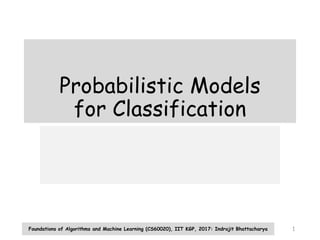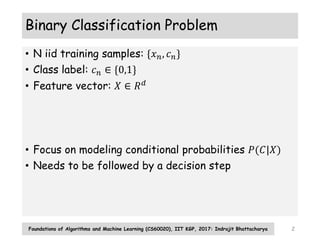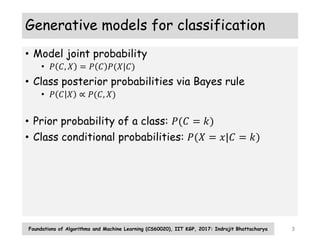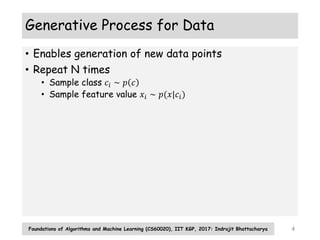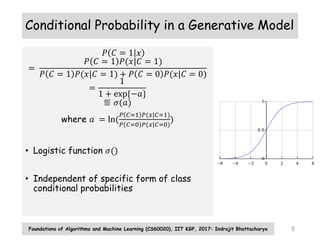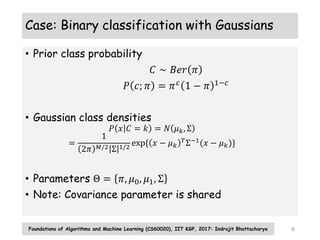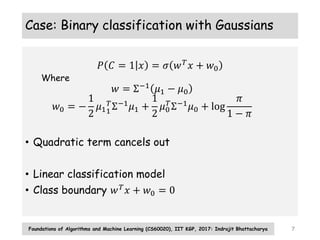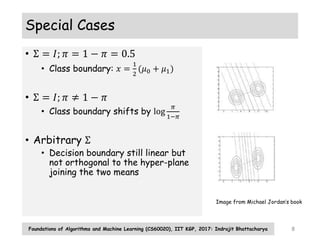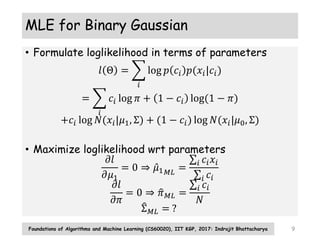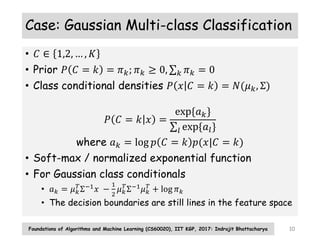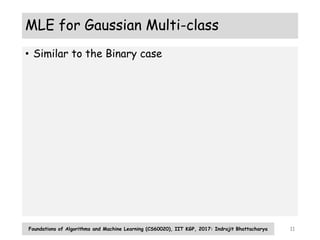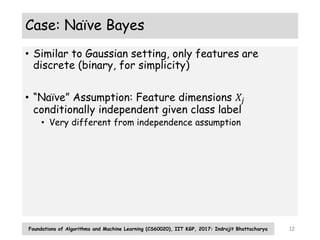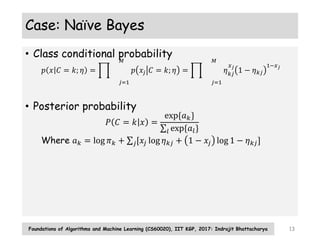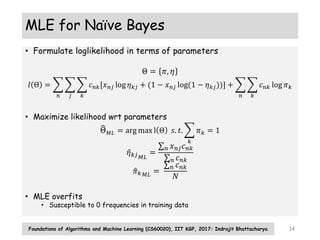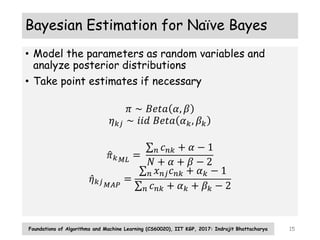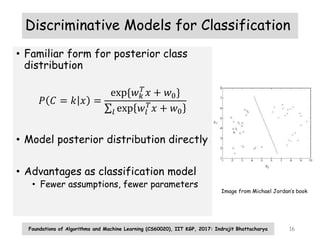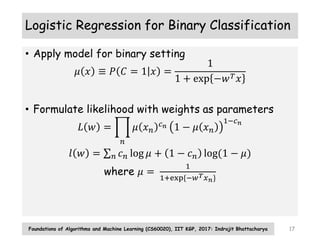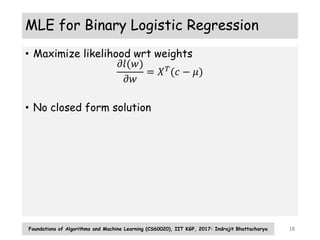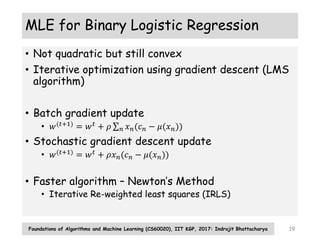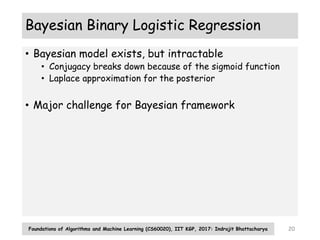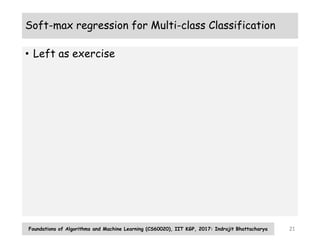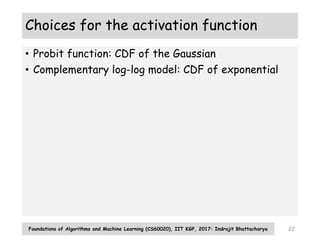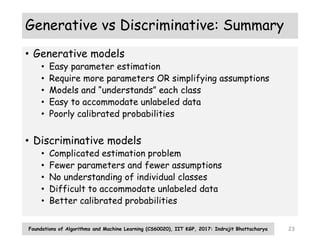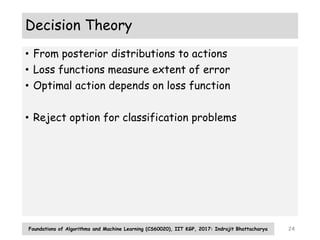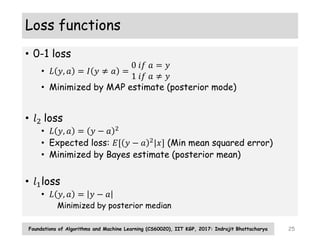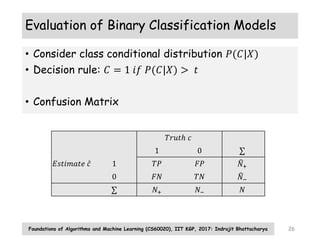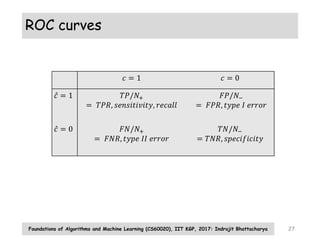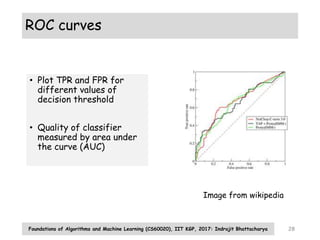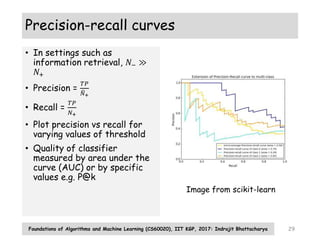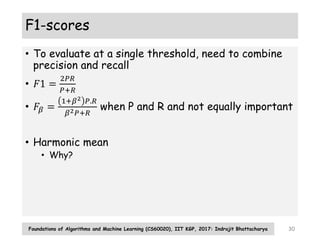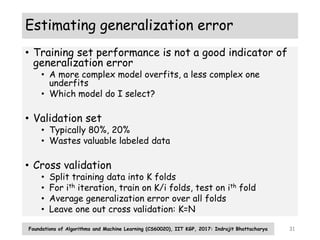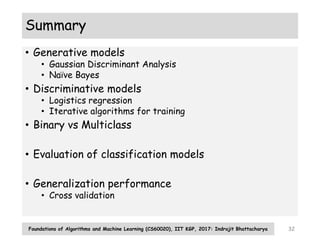1 von 32

### lecture4.pptx

• 1. Probabilistic Models for Classification Foundations of Algorithms and Machine Learning (CS60020), IIT KGP, 2017: Indrajit Bhattacharya 1
• 2. Binary Classification Problem • N iid training samples: {𝑥𝑛, 𝑐𝑛} • Class label: 𝑐𝑛 ∈ {0,1} • Feature vector: 𝑋 ∈ 𝑅𝑑 • Focus on modeling conditional probabilities 𝑃(𝐶|𝑋) • Needs to be followed by a decision step Foundations of Algorithms and Machine Learning (CS60020), IIT KGP, 2017: Indrajit Bhattacharya 2
• 3. Generative models for classification • Model joint probability • 𝑃 𝐶, 𝑋 = 𝑃 𝐶 𝑃(𝑋|𝐶) • Class posterior probabilities via Bayes rule • 𝑃 𝐶 𝑋 ∝ 𝑃(𝐶, 𝑋) • Prior probability of a class: 𝑃(𝐶 = 𝑘) • Class conditional probabilities: 𝑃(𝑋 = 𝑥|𝐶 = 𝑘) Foundations of Algorithms and Machine Learning (CS60020), IIT KGP, 2017: Indrajit Bhattacharya 3
• 4. Generative Process for Data • Enables generation of new data points • Repeat N times • Sample class 𝑐𝑖 ∼ 𝑝 𝑐 • Sample feature value 𝑥𝑖 ∼ 𝑝(𝑥|𝑐𝑖) Foundations of Algorithms and Machine Learning (CS60020), IIT KGP, 2017: Indrajit Bhattacharya 4
• 5. Conditional Probability in a Generative Model 𝑃 𝐶 = 1 𝑥 = 𝑃 𝐶 = 1 𝑃(𝑥|𝐶 = 1) 𝑃 𝐶 = 1 𝑃(𝑥|𝐶 = 1) + 𝑃 𝐶 = 0 𝑃(𝑥|𝐶 = 0) = 1 1 + exp{−𝑎} ≝ 𝜎(𝑎) where 𝑎 = ln( 𝑃 𝐶=1 𝑃(𝑥|𝐶=1) 𝑃 𝐶=0 𝑃(𝑥|𝐶=0) ) • Logistic function 𝜎() • Independent of specific form of class conditional probabilities Foundations of Algorithms and Machine Learning (CS60020), IIT KGP, 2017: Indrajit Bhattacharya 5
• 6. Case: Binary classification with Gaussians • Prior class probability 𝐶 ∼ 𝐵𝑒𝑟 𝜋 𝑃 𝑐; 𝜋 = 𝜋𝑐 1 − 𝜋 1−𝑐 • Gaussian class densities 𝑃 𝑥 𝐶 = 𝑘 = 𝑁 𝜇𝑘, Σ = 1 2𝜋 𝑀/2 Σ 1/2 exp{ 𝑥 − 𝜇𝑘 𝑇Σ−1(𝑥 − 𝜇𝑘)} • Parameters Θ = 𝜋, 𝜇0, 𝜇1, Σ • Note: Covariance parameter is shared Foundations of Algorithms and Machine Learning (CS60020), IIT KGP, 2017: Indrajit Bhattacharya 6
• 7. Case: Binary classification with Gaussians 𝑃 𝐶 = 1 𝑥 = 𝜎 𝑤𝑇 𝑥 + 𝑤0 Where 𝑤 = Σ−1 𝜇1 − 𝜇0 𝑤0 = − 1 2 𝜇11 𝑇Σ−1𝜇1 + 1 2 𝜇0 𝑇 Σ−1𝜇0 + log 𝜋 1 − 𝜋 • Quadratic term cancels out • Linear classification model • Class boundary 𝑤𝑇𝑥 + 𝑤0 = 0 Foundations of Algorithms and Machine Learning (CS60020), IIT KGP, 2017: Indrajit Bhattacharya 7
• 8. Special Cases • Σ = 𝐼; 𝜋 = 1 − 𝜋 = 0.5 • Class boundary: 𝑥 = 1 2 (𝜇0 + 𝜇1) • Σ = 𝐼; 𝜋 ≠ 1 − 𝜋 • Class boundary shifts by log 𝜋 1−𝜋 • Arbitrary Σ • Decision boundary still linear but not orthogonal to the hyper-plane joining the two means Foundations of Algorithms and Machine Learning (CS60020), IIT KGP, 2017: Indrajit Bhattacharya 8 Image from Michael Jordan’s book
• 9. MLE for Binary Gaussian • Formulate loglikelihood in terms of parameters 𝑙 Θ = 𝑖 log 𝑝 𝑐𝑖 𝑝(𝑥𝑖|𝑐𝑖) = 𝑖 𝑐𝑖 log 𝜋 + 1 − 𝑐𝑖 log(1 − 𝜋) +𝑐𝑖 log 𝑁(𝑥𝑖|𝜇1, Σ) + (1 − 𝑐𝑖) log 𝑁(𝑥𝑖|𝜇0, Σ) • Maximize loglikelihood wrt parameters 𝜕𝑙 𝜕𝜇1 = 0 ⇒ 𝜇1𝑀𝐿 = 𝑖 𝑐𝑖𝑥𝑖 𝑖 𝑐𝑖 𝜕𝑙 𝜕𝜋 = 0 ⇒ 𝜋𝑀𝐿 = 𝑖 𝑐𝑖 𝑁 Σ𝑀𝐿 = ? Foundations of Algorithms and Machine Learning (CS60020), IIT KGP, 2017: Indrajit Bhattacharya 9
• 10. Case: Gaussian Multi-class Classification • 𝐶 ∈ 1,2, … , 𝐾 • Prior 𝑃 𝐶 = 𝑘 = 𝜋𝑘; 𝜋𝑘 ≥ 0, 𝑘 𝜋𝑘 = 0 • Class conditional densities 𝑃 𝑥 𝐶 = 𝑘 = 𝑁(𝜇𝑘, Σ) 𝑃 𝐶 = 𝑘 𝑥 = exp{𝑎𝑘} 𝑙 exp{𝑎𝑙} where 𝑎𝑘 = log 𝑝 𝐶 = 𝑘 𝑝(𝑥|𝐶 = 𝑘) • Soft-max / normalized exponential function • For Gaussian class conditionals • 𝑎𝑘 = 𝜇𝑘 𝑇 Σ−1 𝑥 − 1 2 𝜇𝑘 𝑇 Σ−1 𝜇𝑘 𝑇 + log 𝜋𝑘 • The decision boundaries are still lines in the feature space Foundations of Algorithms and Machine Learning (CS60020), IIT KGP, 2017: Indrajit Bhattacharya 10
• 11. MLE for Gaussian Multi-class • Similar to the Binary case Foundations of Algorithms and Machine Learning (CS60020), IIT KGP, 2017: Indrajit Bhattacharya 11
• 12. Case: Naïve Bayes • Similar to Gaussian setting, only features are discrete (binary, for simplicity) • “Naïve” Assumption: Feature dimensions 𝑋𝑗 conditionally independent given class label • Very different from independence assumption Foundations of Algorithms and Machine Learning (CS60020), IIT KGP, 2017: Indrajit Bhattacharya 12
• 13. Case: Naïve Bayes • Class conditional probability 𝑝 𝑥 𝐶 = 𝑘; 𝜂 = 𝑗=1 𝑀 𝑝 𝑥𝑗 𝐶 = 𝑘; 𝜂 = 𝑗=1 𝑀 𝜂𝑘𝑗 𝑥𝑗 1 − 𝜂𝑘𝑗 1−𝑥𝑗 • Posterior probability 𝑃 𝐶 = 𝑘 𝑥 = exp{𝑎𝑘} 𝑙 exp{𝑎𝑙} Where 𝑎𝑘 = log 𝜋𝑘 + 𝑗[𝑥𝑗 log 𝜂𝑘𝑗 + 1 − 𝑥𝑗 log 1 − 𝜂𝑘𝑗] Foundations of Algorithms and Machine Learning (CS60020), IIT KGP, 2017: Indrajit Bhattacharya 13
• 14. MLE for Naïve Bayes • Formulate loglikelihood in terms of parameters Θ = 𝜋, 𝜂 𝑙 Θ = 𝑛 𝑗 𝑘 𝑐𝑛𝑘[𝑥𝑛𝑗 log 𝜂𝑘𝑗 + (1 − 𝑥𝑛𝑗 log(1 − 𝜂𝑘𝑗))] + 𝑛 𝑘 𝑐𝑛𝑘 log 𝜋𝑘 • Maximize likelihood wrt parameters Θ𝑀𝐿 = arg max l Θ 𝑠. 𝑡. 𝑘 𝜋𝑘 = 1 𝜂𝑘𝑗𝑀𝐿 = 𝑛 𝑥𝑛𝑗𝑐𝑛𝑘 𝑛 𝑐𝑛𝑘 𝜋𝑘𝑀𝐿 = 𝑛 𝑐𝑛𝑘 𝑁 • MLE overfits • Susceptible to 0 frequencies in training data Foundations of Algorithms and Machine Learning (CS60020), IIT KGP, 2017: Indrajit Bhattacharya 14
• 15. Bayesian Estimation for Naïve Bayes • Model the parameters as random variables and analyze posterior distributions • Take point estimates if necessary 𝜋 ∼ 𝐵𝑒𝑡𝑎 𝛼, 𝛽 𝜂𝑘𝑗 ∼ 𝑖𝑖𝑑 𝐵𝑒𝑡𝑎 𝛼𝑘, 𝛽𝑘 𝜋𝑘𝑀𝐿 = 𝑛 𝑐𝑛𝑘 + 𝛼 − 1 𝑁 + 𝛼 + 𝛽 − 2 𝜂𝑘𝑗𝑀𝐴𝑃 = 𝑛 𝑥𝑛𝑗𝑐𝑛𝑘 + 𝛼𝑘 − 1 𝑛 𝑐𝑛𝑘 + 𝛼𝑘 + 𝛽𝑘 − 2 Foundations of Algorithms and Machine Learning (CS60020), IIT KGP, 2017: Indrajit Bhattacharya 15
• 16. Discriminative Models for Classification • Familiar form for posterior class distribution 𝑃 𝐶 = 𝑘 𝑥 = exp{𝑤𝑘 𝑇 𝑥 + 𝑤0} 𝑙 exp 𝑤𝑙 𝑇 𝑥 + 𝑤0 • Model posterior distribution directly • Advantages as classification model • Fewer assumptions, fewer parameters Foundations of Algorithms and Machine Learning (CS60020), IIT KGP, 2017: Indrajit Bhattacharya 16 Image from Michael Jordan’s book
• 17. Logistic Regression for Binary Classification • Apply model for binary setting 𝜇 𝑥 ≡ 𝑃 𝐶 = 1 𝑥 = 1 1 + exp −𝑤𝑇𝑥 • Formulate likelihood with weights as parameters 𝐿 𝑤 = 𝑛 𝜇 𝑥𝑛 𝑐𝑛 1 − 𝜇 𝑥𝑛 1−𝑐𝑛 𝑙 𝑤 = 𝑛 𝑐𝑛 log 𝜇 + 1 − 𝑐𝑛 log(1 − 𝜇) where 𝜇 = 1 1+exp{−𝑤𝑇𝑥𝑛} Foundations of Algorithms and Machine Learning (CS60020), IIT KGP, 2017: Indrajit Bhattacharya 17
• 18. MLE for Binary Logistic Regression • Maximize likelihood wrt weights 𝜕𝑙(𝑤) 𝜕𝑤 = 𝑋𝑇 (𝑐 − 𝜇) • No closed form solution Foundations of Algorithms and Machine Learning (CS60020), IIT KGP, 2017: Indrajit Bhattacharya 18
• 19. MLE for Binary Logistic Regression • Not quadratic but still convex • Iterative optimization using gradient descent (LMS algorithm) • Batch gradient update • 𝑤(𝑡+1) = 𝑤𝑡 + 𝜌 𝑛 𝑥𝑛(𝑐𝑛 − 𝜇(𝑥𝑛)) • Stochastic gradient descent update • 𝑤(𝑡+1) = 𝑤𝑡 + 𝜌𝑥𝑛(𝑐𝑛 − 𝜇(𝑥𝑛)) • Faster algorithm – Newton’s Method • Iterative Re-weighted least squares (IRLS) Foundations of Algorithms and Machine Learning (CS60020), IIT KGP, 2017: Indrajit Bhattacharya 19
• 20. Bayesian Binary Logistic Regression • Bayesian model exists, but intractable • Conjugacy breaks down because of the sigmoid function • Laplace approximation for the posterior • Major challenge for Bayesian framework Foundations of Algorithms and Machine Learning (CS60020), IIT KGP, 2017: Indrajit Bhattacharya 20
• 21. Soft-max regression for Multi-class Classification • Left as exercise Foundations of Algorithms and Machine Learning (CS60020), IIT KGP, 2017: Indrajit Bhattacharya 21
• 22. Choices for the activation function • Probit function: CDF of the Gaussian • Complementary log-log model: CDF of exponential Foundations of Algorithms and Machine Learning (CS60020), IIT KGP, 2017: Indrajit Bhattacharya 22
• 23. Generative vs Discriminative: Summary • Generative models • Easy parameter estimation • Require more parameters OR simplifying assumptions • Models and “understands” each class • Easy to accommodate unlabeled data • Poorly calibrated probabilities • Discriminative models • Complicated estimation problem • Fewer parameters and fewer assumptions • No understanding of individual classes • Difficult to accommodate unlabeled data • Better calibrated probabilities Foundations of Algorithms and Machine Learning (CS60020), IIT KGP, 2017: Indrajit Bhattacharya 23
• 24. Decision Theory • From posterior distributions to actions • Loss functions measure extent of error • Optimal action depends on loss function • Reject option for classification problems Foundations of Algorithms and Machine Learning (CS60020), IIT KGP, 2017: Indrajit Bhattacharya 24
• 25. Loss functions • 0-1 loss • 𝐿 𝑦, 𝑎 = 𝐼 𝑦 ≠ 𝑎 = 0 𝑖𝑓 𝑎 = 𝑦 1 𝑖𝑓 𝑎 ≠ 𝑦 • Minimized by MAP estimate (posterior mode) • 𝑙2 loss • 𝐿 𝑦, 𝑎 = 𝑦 − 𝑎 2 • Expected loss: 𝐸[ 𝑦 − 𝑎 2|𝑥] (Min mean squared error) • Minimized by Bayes estimate (posterior mean) • 𝑙1loss • 𝐿 𝑦, 𝑎 = 𝑦 − 𝑎 Minimized by posterior median Foundations of Algorithms and Machine Learning (CS60020), IIT KGP, 2017: Indrajit Bhattacharya 25
• 26. Evaluation of Binary Classification Models • Consider class conditional distribution 𝑃(𝐶|𝑋) • Decision rule: 𝐶 = 1 𝑖𝑓 𝑃(𝐶|𝑋) > 𝑡 • Confusion Matrix Foundations of Algorithms and Machine Learning (CS60020), IIT KGP, 2017: Indrajit Bhattacharya 26 𝑇𝑟𝑢𝑡ℎ 𝑐 1 0 𝐸𝑠𝑡𝑖𝑚𝑎𝑡𝑒 𝑐 1 𝑇𝑃 𝐹𝑃 𝑁+ 0 𝐹𝑁 𝑇𝑁 𝑁− 𝑁+ 𝑁− 𝑁
• 27. ROC curves Foundations of Algorithms and Machine Learning (CS60020), IIT KGP, 2017: Indrajit Bhattacharya 27 𝑐 = 1 𝑐 = 0 𝑐 = 1 𝑇𝑃/𝑁+ = 𝑇𝑃𝑅, 𝑠𝑒𝑛𝑠𝑖𝑡𝑖𝑣𝑖𝑡𝑦, 𝑟𝑒𝑐𝑎𝑙𝑙 𝐹𝑃/𝑁− = 𝐹𝑃𝑅, 𝑡𝑦𝑝𝑒 𝐼 𝑒𝑟𝑟𝑜𝑟 𝑐 = 0 𝐹𝑁/𝑁+ = 𝐹𝑁𝑅, 𝑡𝑦𝑝𝑒 𝐼𝐼 𝑒𝑟𝑟𝑜𝑟 𝑇𝑁/𝑁− = 𝑇𝑁𝑅, 𝑠𝑝𝑒𝑐𝑖𝑓𝑖𝑐𝑖𝑡𝑦
• 28. ROC curves • Plot TPR and FPR for different values of decision threshold • Quality of classifier measured by area under the curve (AUC) Foundations of Algorithms and Machine Learning (CS60020), IIT KGP, 2017: Indrajit Bhattacharya 28 Image from wikipedia
• 29. Precision-recall curves • In settings such as information retrieval, 𝑁− ≫ 𝑁+ • Precision = 𝑇𝑃 𝑁+ • Recall = 𝑇𝑃 𝑁+ • Plot precision vs recall for varying values of threshold • Quality of classifier measured by area under the curve (AUC) or by specific values e.g. P@k Foundations of Algorithms and Machine Learning (CS60020), IIT KGP, 2017: Indrajit Bhattacharya 29 Image from scikit-learn
• 30. F1-scores • To evaluate at a single threshold, need to combine precision and recall • 𝐹1 = 2𝑃𝑅 𝑃+𝑅 • 𝐹𝛽 = 1+𝛽2 𝑃.𝑅 𝛽2𝑃+𝑅 when P and R and not equally important • Harmonic mean • Why? Foundations of Algorithms and Machine Learning (CS60020), IIT KGP, 2017: Indrajit Bhattacharya 30
• 31. Estimating generalization error • Training set performance is not a good indicator of generalization error • A more complex model overfits, a less complex one underfits • Which model do I select? • Validation set • Typically 80%, 20% • Wastes valuable labeled data • Cross validation • Split training data into K folds • For ith iteration, train on K/i folds, test on ith fold • Average generalization error over all folds • Leave one out cross validation: K=N Foundations of Algorithms and Machine Learning (CS60020), IIT KGP, 2017: Indrajit Bhattacharya 31
• 32. Summary • Generative models • Gaussian Discriminant Analysis • Naïve Bayes • Discriminative models • Logistics regression • Iterative algorithms for training • Binary vs Multiclass • Evaluation of classification models • Generalization performance • Cross validation Foundations of Algorithms and Machine Learning (CS60020), IIT KGP, 2017: Indrajit Bhattacharya 32